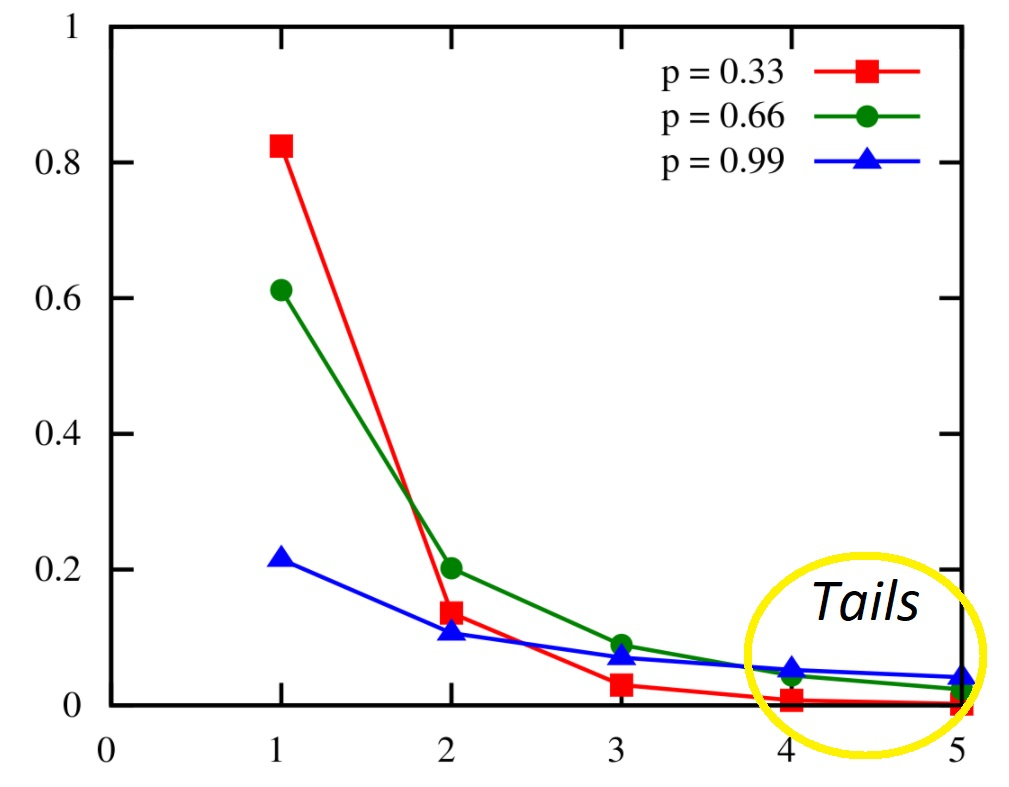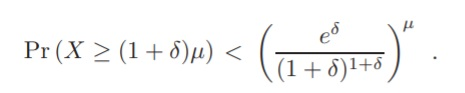# Tail Bound: Definition, Examples

Share on

## What is a Tail Bound?

The tails of a random variable X are those parts of the probability mass function far from the mean .Sometimes we want to create tail bounds (or tail inequalities) on the PMF, or bound the probability that the random variable deviates a long way from the mean. For example, if the PMF represents a budget, we might not want to go over that budget by a factor of 3. Or, if I expect 10,000 people with student loans are in default, I might want to know the probability a million borrowers will default.

## Types of Tail Bound

Various formulas exist for tail bounds. One way to place a tail bound is by controlling the moments of the random variable X.

Markov’s inequality is the simplest tail bound, only requiring the existence of the first moment. It states that, for a nonnegative random variable X with mean μ = εX ,

Pr(X ≥ k) ≤ μ/k.

Although simple, the bounds that Markov’s inequality implies are usually not useful because they are too weak.

The Chebyshev bound is slightly stronger than Markov’s inequality. It is defined for a random variable X with mean μ = εX with standard deviation σ = √(ε((X – μ)2)) for any δ ≥ 1:

Pr(|X – μ ≥ δσ) ≤ δ-2.

One of the more complex tail bounds is the Chernoff bound, which requires that the moment generating function exists. For many random variables, this requirement is usually not a problem because the MGF will exist in a neighborhood around 0 . The Chernoff bound has several equivalent forms. One form is for Poisson trials Xi with sum X = ΣiXi and mean μ = εX, for any δ > 0:## References

PMF Image: Qwfp, CC BY-SA 3.0 , via Wikimedia Commons
 Tail bounds. Retrieved November 28, 2021 from: https://courses.cs.washington.edu/courses/cse312/11au/slides/09tails.pdf
 Supplementary Lecture I: Tail Bounds. Retrieved November 28, 2021 from: http://www.cs.cornell.edu/courses/cs681/2007fa/Handouts/tailBounds.pdf
 36-705: Intermediate Statistics Fall 2019

CITE THIS AS:
Stephanie Glen. "Tail Bound: Definition, Examples" From StatisticsHowTo.com: Elementary Statistics for the rest of us! https://www.statisticshowto.com/tail-bound/
---------------------------------------------------------------------------Need help with a homework or test question? With Chegg Study, you can get step-by-step solutions to your questions from an expert in the field. Your first 30 minutes with a Chegg tutor is free!